# Binary to Decimal and Decimal to Binary Conversion (Examples Included)

The number system that we are mostly familiar with is a decimal number system. The decimal number system has a base of ten which implies that there are ten numbers by the help of which we can represent any number of the decimal family. The numbers are from 0 to 9. Representation is such as (15)10 the base 10 is written as suffix or radix. If it is not written then by default we must understand that it is a decimal number by default.

On the other hand, binary number invented by Gottfried Leibniz in 1679 has a base of two. “Bi” means two so from there we can say that the base of the binary system is 2. That is, only two numbers are sufficient to represent a number in binary format. The numbers which are used in binary number system are 0 and 1.

Binary number can be represented by putting 2 in the prefix which denotes the base. If the base is not given, then it is by default assumed to be a decimal number .We have to be very careful in writing a binary number, a slight mistake may result in a very serious error. For example, a binary number is written as follows: (00110)2.

Now a question may arise, why do we need binary number? We have decimal number system which is familiar to all of us and most of the persons do not understand binary. The answer is that any programmable device or a processor can work in two modes either high or low.

Here, high denotes the supply is connected to that point and low denotes that the point is grounded or is at a state of zero volts. This is called positive logic and in other logic system the reverse is taken which is known as negative logic system.

Also, we can say that a high means that it performs some function or work and low indicates that it has not performed any work. The reverse may also be true if we take negative logic system.

So, from the above description we can say that it is much easier and convenient to use binary number system in the computer instead of decimal and also conversion will be required in order that the output result which is given in binary form should be converted to decimal for the sake of the user.

## Binary to Decimal Conversion

### Conversion of Integer Numbers

Just as you can convert any binary numbers to hexadecimal, or convert binary numbers to octal, any number in the binary number system can be converted into the hexadecimal number system.

This conversion is also very simple method. Let, a binary number be (11010)2 , where the weight of the binary digits from most significant bit are 24, 23, 22, 21, 20 respectively.

Now the bits are multiplied with their weights, and the sum of those products is the respective decimal number. Now let us follow the following steps mathematically: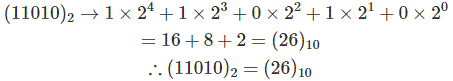Hence, (26)10 is the required decimal number. This is how binary to decimal conversion is performed.

As another example, we convert the binary number (1110)2 to a decimal number: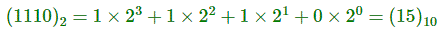### Conversion of Decimal Point Number to Decimal

This can also be done in the same way, however after the decimal point the number should be multiplied with 2-1, 2-2 etc.
For example,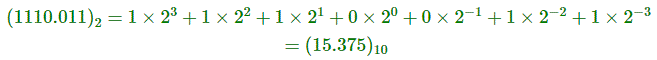## Decimal to Binary Conversion

### Integer Decimal Numbers to Binary

Divide the number by 2 and take only the remainder, if division is completed than take only the remainder which gives the binary number.

Suppose we are converting the decimal number (87)10. We divide 87 by 2 and get 43 as the quotient and 1 as the remainder. These remainders are written beside as shown below.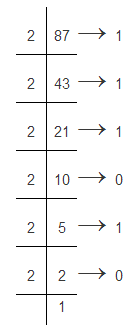The possibility of remainder (87)10 = (1010111)2 is only 1 and 0. Thus the number is counted from the last remainder. Such as 1 0 1 0 1 1 1. This is how decimal to binary conversion is done.

### Fraction Decimal Numbers to Binary

In this case, the successive multiplication is done. The number which is to be converted is multiplied with base or radix of binary number which is 2. The integer part or the carry of the product is taken out and the same process is repeated until we get an integer. For example-
The binary equivalent of (.95)10 is evaluated as follows-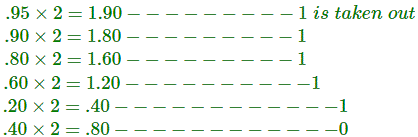Since, we are not getting the integer value after successive multiplication, we can approximate the value to be (.111110….)2.

## Negative Decimal to Binary

In case of a negative number we can go for 2’s complement representation of a signed number.
Example- 9 = 0000 1001
1’s complement = 1111 0110.
Adding 1 we get = 11110111 which is the 2’s complement representation of (-9).

Want To Learn Faster? 🎓
Get electrical articles delivered to your inbox every week.
No credit card required—it’s 100% free.